## Sunday, January 29, 2012

### New version of plotSimmap that can plot node numbers

I just posted a new version of plotSimmap (direct link to code here) which can also plot node numbers (that is, the numbers in the matrix \$edge for all non-terminal nodes). These are the same labels used by nodelabels in ape if no node names have been assigned.

This was pretty easy. I just plotted a rectangle with white background (using the graphics function symbols) at the end of each branch not ending in a tip. (I have to figure out where these branches go anyway, so the problem of identifying the vertical position is already solved.) I specified the height & width of box to be slightly larger than the label text length & width using strheight and strwidth. I then wrote inside the box using text.

The result can be visualized by doing something like the following:

require(phytools)
source("plotSimmap.R") # load the new version of plotSimmap
Q<-matrix(c(-1,1,1,-1),2,2)
cols<-c("blue","red"); names(cols)<-c(1,2)
tree<-sim.history(pbtree(n=25,scale=1),Q,anc=1)
plotSimmap(tree,cols,lwd=3,pts=F,node.numbers=T)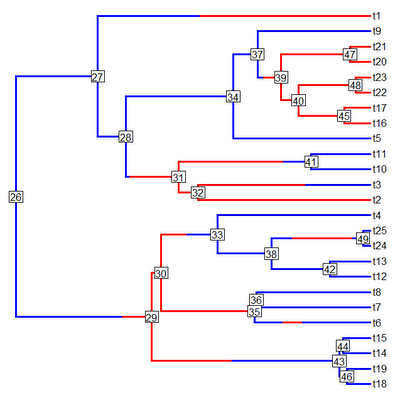That's pretty cool. Since plotTree is now just a wrapper for plotSimmap with a really simple change to plotTree (code here) you can now visualize node numbers using that function as well (although this is pretty much completely redundant with plot.phylo and nodelabels in ape).

source("plotTree.R") # load the new version of plotTree
plotTree(tree,pts=F,lwd=3,node.numbers=T)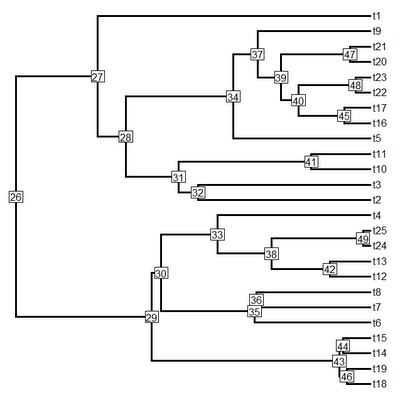That's it!

## Friday, January 27, 2012

### Ottawa SSB symposiumThe speaker line-up for the SSB symposium I am co-organizing with Cécile Ané has been posted online on the Evolution 2012 website here.

New phylogenetic methods for quantitative trait evolution

Organizers: Cécile Ané (University of Wisconsin-Madison) and Liam J. Revell (University of Massachusetts Boston)

Speakers:
· Cécile Ané (University of Wisconsin)
· Carl Boettiger (University of California, Davis)
· Joseph Felsenstein (University of Washington)
· Brian C. O'Meara (University of Tennessee)
· Liam J. Revell (University of Massachusetts)
· Lars Schmitz (University of California, Davis)

Should be fun!

### New function to paint sub-trees with different states

I just posted a new function to "paint" subtrees on a phylogeny with different mapped states. I did this in response to a user request and the purpose is to allow users to specify arbitrary mapping for use in functions like brownie.lite, brownieREML, and evol.vcv. The name of this new function is paintSubTree and a direct link to the function code is here.

The way this function works is pretty straightforward. Basically, it takes the tree & the basal node number of a sub-tree on the phylogeny. It then paints all the tipward edges of that node (as well as the stem, if selected) in an arbitrarily specified state. Since the function also retains any existing mapping, it can be used multiple times to paint different clades, and even nested clades, in different states.

I accomplished this by first using a function called getDescendants (basically the same as phangorn:Descendants) to compute all the descendant node and tip numbers from the target node. Then I use this set of node numbers (and the handy base function which) to match the tipward nodes to the \$edge matrix and then paint the branches accordingly.

Here is a quick illustration of use. First, let's simulate a small tree, set the tip & tip numbers equal, and then plot the tree with node numbers:

> require(phytools)
> tree<-pbtree(n=15); tree\$tip.label<-1:15
> plot(tree,font=1); nodelabels(bg="white")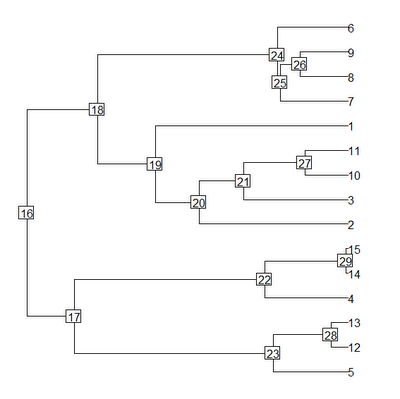Now let's paint all the edges from node 20 in a derived state, including the stem leading to node 20:

> tree<-paintSubTree(tree,node=20,state="2",stem=T)
> cols<-c("blue","red","green"); names(cols)<-c(1,2,3)
> plotSimmap(tree,cols,lwd=3,pts=F)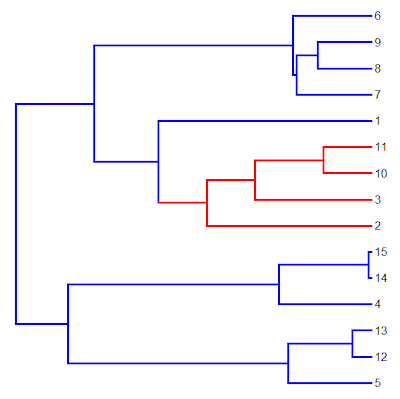Mission accomplished. Now, let's paint a separate part of the tree, say the clade descending from node 24 (this time excluding the stem), with state 3:

> tree<-paintSubTree(tree,node=24,state="3",stem=F)
> plotSimmap(tree,cols,lwd=3,pts=F)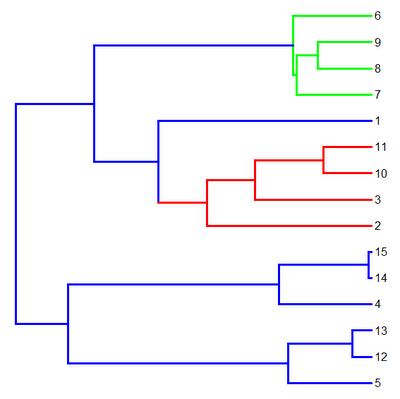Finally, we can also paint nested clades as well as only tip edges. If we paint a tip edge only, obviously stem must be set to TRUE. Let's do that:

> tree<-paintSubTree(tree,node=3,state="3",stem=T)
> plotSimmap(tree,cols,lwd=3,pts=F)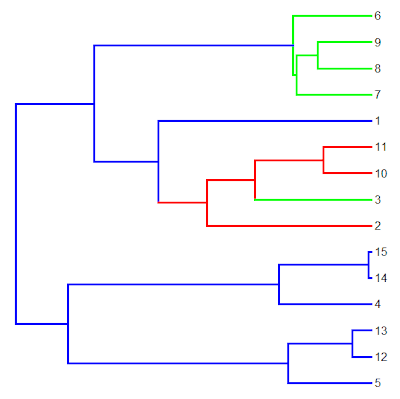Cool.

## Thursday, January 26, 2012

### Function to get descendant node numbers

So, I'm working on a different project that required me to write a function to pull out the descendant node (& tip) numbers from any internal node on the tree. Even though I feel like I've done this before, I struggled coming up with something that made sense. This is what I ended up with:

getDescendants<-function(tree,node,curr=NULL){
if(is.null(curr)) curr<-vector()
daughters<-tree\$edge[which(tree\$edge[,1]==node),2]
curr<-c(curr,daughters)
w<-which(daughters>=length(tree\$tip))
if(length(w)>0) for(i in 1:length(w))
curr<-getDescendants(tree,daughters[w[i]],curr)
return(curr)
}

Here's a quick example of how it works:

> tree<-pbtree(n=10)
> tree\$tip.label<-1:10
> # this lines up tip numbers & labels for simplicity
> par(mar=c(1,1,1,1)) # set margins
> plot(tree,font=1); nodelabels(bg="white")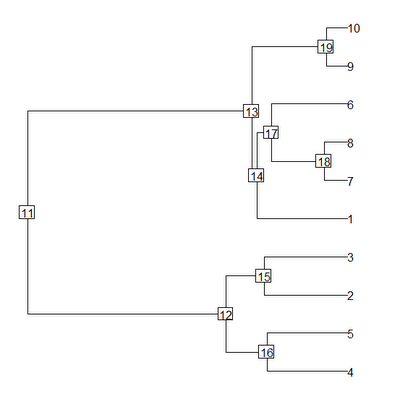Now we can pull the numbers for the descendant nodes & tips for any internal node. For instance:

> getDescendants(tree,node=17)
 18 6 7 8
> getDescendants(tree,node=12)
 16 15 4 5 2 3
> getDescendants(tree,node=19)
 9 10

Anyway, seems to work, but if anyone is aware of an existing function for this, please pass it along.

### Getting tip states from sim.history

A user just emailed me to ask how you get the tip states from a character history simulated using sim.history. This is pretty easy because sim.history stores them in the vector \$states.

So, for one tree:

> require(phytools)
> tree<-pbtree(n=25,scale=1)
> Q<-matrix(c(-1,1,1,-1),2,2)
> mtree<-sim.history(tree,Q)
> mtree\$states
t1  t2  t3  t4  t5  t6  t7  t8  t9 t10 t11 t12 t13 t14 t15 t16
"2" "1" "2" "2" "2" "2" "2" "1" "2" "2" "2" "2" "2" "2" "1" "2"
t17 t18 t19 t20 t21 t22 t23 t24 t25
"2" "2" "2" "1" "2" "2" "2" "2" "2"

Or, for multiple trees:

> mtrees<-sim.history(tree,Q,nsim=10)
> mtrees[]\$states # first simulated history
t1  t2  t3  t4  t5  t6  t7  t8  t9 t10 t11 t12 t13 t14 t15 t16
"1" "2" "1" "2" "2" "2" "2" "2" "1" "1" "2" "1" "1" "1" "1" "1"
t17 t18 t19 t20 t21 t22 t23 t24 t25
"1" "1" "1" "1" "2" "1" "1" "1" "1"
> mtrees[]\$states # second history
t1  t2  t3  t4  t5  t6  t7  t8  t9 t10 t11 t12 t13 t14 t15 t16
"2" "1" "1" "2" "2" "2" "1" "1" "1" "2" "1" "1" "1" "2" "2" "2"
t17 t18 t19 t20 t21 t22 t23 t24 t25
"1" "1" "1" "2" "1" "1" "1" "1" "1"
> # etc, or to get all states from all simulated histories (in columns)
> X<-sapply(mtrees,function(x) x\$states) # all histories
> X
[,1] [,2] [,3] [,4] [,5] [,6] [,7] [,8] [,9] [,10]
t1  "1"  "2"  "2"  "1"  "2"  "1"  "2"  "1"  "1"  "2"
t2  "2"  "1"  "1"  "2"  "2"  "2"  "1"  "1"  "2"  "2"
t3  "1"  "1"  "2"  "2"  "1"  "1"  "2"  "2"  "2"  "1"
...

That's it!

## Tuesday, January 17, 2012

### Phytools demo vignette

I'm down in Puerto Rico on a lab field trip right now, but while in flight I prepared my recent mini-workshop on phytools that I gave at UCLA in the format of an R vignette. Keep in mind that this demo highlights only a small subset of the capabilities of the phytools package. I have also posted the file to scribd and you can view the embedded PDF below:

Phytools Demo

I apologize that this is a little rough around the edges, but it is my first R vignette and evidently some of the workshop attendees were interested in getting my notes so I wanted to post these as quickly as possible.

## Monday, January 16, 2012

### Fitting Pagel's λ for a single trait using multiple methods

Because it related to something else I was working on, I today did a quick comparison of the (at least 4) different methods available in R to fit Pagel's λ model for a single trait. I just did this for a single 200 taxon tree and compared the estimates (which are all, to reasonable numerical precision, the same) and the computation time. A more thorough comparison would require that we run this code over multiple simulations to try and determine the robustness of each function. This I have not done, but anyone wishing to loop the following analyses 100 or more times should be my guest. This was run on my VAIO AMD E-450 laptop @ 1.65 GHz (so, not very fast):

> require(phytools); require(geiger); require(caper); require(nlme)
> tree<-pbtree(n=200,scale=1) # simulate tree
> x<-fastBM(lambdaTree(tree,0.7)) # simulate data
> # fit using phytools:phylosig
> system.time(m1<-phylosig(tree,x,method="lambda"))
user  system elapsed
2.79    0.00    2.79
> # fit using geiger:fitContinuous
> system.time(m2<-fitContinuous(tree,x,model="lambda"))
Fitting  lambda model:
user  system elapsed
137.11    0.42  138.90
> # fit using nlme:gls
> d<-data.frame(x)
> system.time(m3<-gls(x~1,data=d,correlation=corPagel(0.5,tree,fixed=F), method="ML"))
user  system elapsed
51.56    0.22   53.86
> # fit using caper:pgls
> d<-data.frame(x,names(x)); colnames(d)<-"Species"
> system.time(m4<-pgls(x~1,comparative.data(tree,d,"Species"), lambda="ML"))
user  system elapsed
38.13    0.01   38.25

We can then get a summary as follows:

> result<-matrix(c(m1\$lambda,m1\$logL,
m2\$Trait1\$lambda,m2\$Trait1\$lnl,
m3\$modelStruct\$corStruct,m3\$logLik,
m4\$param["lambda"],m4\$model\$log.lik),4,2,
dimnames=list(c("phylosig","fitContinuous","gls","pgls"),
c("lambda","logL")),byrow=T)
> result
lambda      logL
phylosig      0.7478915 -230.7348
fitContinuous 0.7478919 -230.7348
gls           0.7478922 -230.7348
pgls          0.7478922 -230.7348

So, at least for this example, phylosig is quite a bit faster, but it makes no discernible difference in the results. Why this would be the case is not at all clear to me (phylosig uses the built in optimizer, optimize, and performs full ML not REML), but I hope that it does not come at the cost of robust estimation. Certainly this could be assessed by repeating the above analysis for many simulations.

## Tuesday, January 10, 2012

### New version of phytools (v0.1-6)

I just posted a new version of phytools (phytools_0.1-6) to the phytools homepage. I have also submitted this version to CRAN.

Some of the updates in this version:

1) New function sampleFrom which takes a vector of means, a vector of variances, and a vector of sample sizes (or a range on which to pick random sample sizes); and then it generates a vector containing random samples from each population (described here). This is useful for simulating data to be run through the function fitBayes.

2) A new version of the tree plotting function plotTree. This version really just acts as a wrapper for plotSimmap so that it can use the options of plotSimmap, without a mapped trait.

3) A new version of phylosig which also returns the log-likelihood for λ=0.0 if phylosig(...,method="lambda",test=TRUE). This version also has better error checking and new internal functions to match the data (and, optionally, the standard errors) to the tree (described here). These updates were added by user request.

4) A new function for stochastic Yule trees with various options called pbtree (here).

5) A new version of phenogram that can also color the edges by a discrete character mapping on the tree (here).

6) Finally, I new function evolvcv.lite that can be used to fit common correlation models and common rate models for the method of evol.vcv (here).

## Saturday, January 7, 2012

### SICB talk 2012

Today I gave a talk at the Society for Integrative and Comparative Biology (i.e., SICB) meeting in Charleston, SC. I talked about two things: 1) I dissected the data analysis pipeline in which we use stochastic character mapping to test the hypothesis that the state of a discrete character influences the rate of a continuous trait; and 2) I presented the Bayesian MCMC method for comparative analysis with intraspecific variation (as implemented in fitBayes). Anyone who has seen me give a talk knows that I generally put very little text on my powerpoint slides; however, I have posted the slides of my presentation (in pdf format) below anyway, because I used a format in which the analysis code (wherever possible) is pasted in a text box below the data figures and results. I thought that some of the readers of the blog might be interested in these slides and code. Enjoy!
Revell.SICB

## Friday, January 6, 2012

### New function to sample from normal (or other types of) distributions

I just wrote a new function to generate a set of random data for individual observations as if they were drawn from a set of univariate normal distributions with species specific means and variances specified in a vector of means & variances. This is to automate the data simulation process for the fitBayes (e.g., see here). I have not yet posted this only, but the function is as follows:

sampleFrom<-function(xbar=0,xvar=1,n=1,randn=NULL,type="norm"){
if(length(xvar)==1&&length(xbar)!=length(xvar))
xvar<-rep(xvar,length(xbar))
if(!is.null(randn))
for(i in 1:length(xbar))
n[i]<-floor(runif(n=1,min=randn,max=(randn+1)))
x<-vector()
for(i in 1:length(xbar)){
y<-rnorm(n=n[i],mean=xbar[i],sd=sqrt(xvar[i]))
names(y)<-rep(names(xbar)[i],length(y))
x<-c(x,y)
}
return(x)
}

Seems to work for what I'm trying to do. Let me know if you see any bugs.

## Wednesday, January 4, 2012

### MCMC method article in print!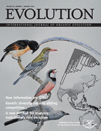I have just been notified that the paper describing our Bayesian MCMC method for identifying exceptional phenotypic evolution (implemented in the phytools function evol.rate.mcmc) is now finally in print at Evolution. I wrote several prior blog posts describing this method as well as some of the challenges involved in averaging our samples from the posterior distribution (e.g., 1, 2, 3, 4, 5, 6, 7, 8, 9). My coauthors for this article were Luke Mahler, Pedro Peres-Neto, and Ben Redelings. Ben, who is a real biomathematician, was my office mate at NESCent and was really instrumental in helping me figure out some of the key problems of this method. The paper is available here, so please check it out (and don't hesitate to email me if your institution does not subscribe to the journal).